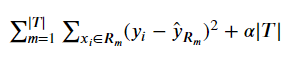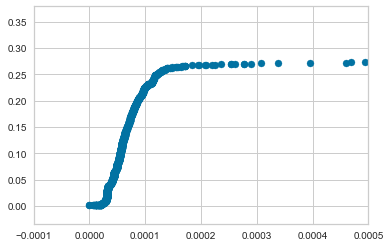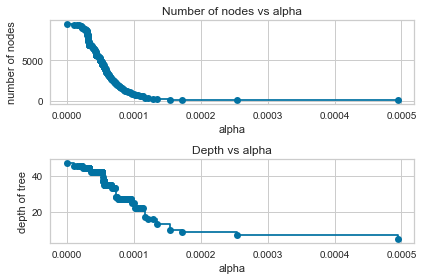# Decision Tree Pruning

As mentioned in our notebook on Decision Trees we can apply hard stops such as max_depth, max_leaf_nodes, or min_samples_leaf to enforce hard-and-fast rules we employ when fitting our Decision Trees to prevent them from growing unruly and thus overfitting.

Alternatively, Chapter 8 of ISL proposes a process called Cost Complexity Pruning, which acts as a sort of countermeasure for paring down a large tree that was trained more-or-less unpenalized. The method employs a constant alpha that penalizes our cost function for each of our terminal nodes for a given tree, denoted as |T|.

The Regression case looks like:

from IPython.display import Image

Image('images/regression_cost_prune.PNG')(Obviously, the Classification has the same term at the end, just a more-appropriate loss function.)

They go on to state an interesting property of this form

As we increase alpha from zero, branches get pruned from the tree in a predictable fashion, so obtaining the whole sequence of subtrees as a function of alpha is easy.

I really like this video’s explanation of that intuition.

## In scikit-learn

Note: This section is only relevant as of version 0.22.

At the time of writing this, the docs regarding this feature aren’t live yet, so I’ll borrow heavier than usual in lieu of being able to link a permanent URL.

Otherwise, let’s jump into it.

We draw on a sample dataset used for classification problems

%pylab inline

X, y = load_credit()
Populating the interactive namespace from numpy and matplotlib

X.head()
limit sex edu married age apr_delay may_delay jun_delay jul_delay aug_delay ... jun_bill jul_bill aug_bill sep_bill apr_pay may_pay jun_pay jul_pay aug_pay sep_pay
0 20000 2 2 1 24 2 2 -1 -1 -2 ... 689 0 0 0 0 689 0 0 0 0
1 120000 2 2 2 26 -1 2 0 0 0 ... 2682 3272 3455 3261 0 1000 1000 1000 0 2000
2 90000 2 2 2 34 0 0 0 0 0 ... 13559 14331 14948 15549 1518 1500 1000 1000 1000 5000
3 50000 2 2 1 37 0 0 0 0 0 ... 49291 28314 28959 29547 2000 2019 1200 1100 1069 1000
4 50000 1 2 1 57 -1 0 -1 0 0 ... 35835 20940 19146 19131 2000 36681 10000 9000 689 679

5 rows × 23 columns

And we can just sausage it right into a Decision Tree with sklearn

from sklearn.tree import DecisionTreeClassifier

clf = DecisionTreeClassifier(random_state=0)
clf.fit(X, y)
DecisionTreeClassifier(ccp_alpha=0.0, class_weight=None, criterion='gini',
max_depth=None, max_features=None, max_leaf_nodes=None,
min_impurity_decrease=0.0, min_impurity_split=None,
min_samples_leaf=1, min_samples_split=2,
min_weight_fraction_leaf=0.0, presort='deprecated',
random_state=0, splitter='best')


And if we fit it with the default arguments, we’d wind up getting a tree that had 4750 terminal nodes

clf.get_n_leaves()
4750


On the otherhand, if we re-instantiated clf as a blank DecisionTreeClassifier

clf = DecisionTreeClassifier(random_state=0)

And called .cost_complexity_pruning_path() instead of fit()

path = clf.cost_complexity_pruning_path(X, y)

Behind the scenes this actually fits the generic Decision Tree, then iteratively ratchets up our alpha value and aggregates the impurities of each terminal node. The path variable gets loaded with arrays ccp_alphas and impurities– the values of alpha that cause changes in the impurities and their corresponding results.

path.ccp_alphas
array([0.00000000e+00, 0.00000000e+00, 6.48148148e-06, ...,
3.34121368e-03, 1.03500728e-02, 5.24158517e-02])

path.impurities
array([0.00074444, 0.00074444, 0.00076389, ..., 0.2817752 , 0.29212527,
0.34454112])


We wind up finding 1899 different values for alpha

len(path.ccp_alphas)
1889


And look at how sensitive impurity is to different alpha values– check that x-scale!

fix, ax = plt.subplots()
ax.scatter(path.ccp_alphas, path.impurities)
ax.set_xlim([-0.0001, 0.0005])
(-0.0001, 0.0005)To cement our intuition here, let’s train a couple hundred Decision Trees using increasing values of alpha

clfs = []
for ccp_alpha in path.ccp_alphas[::10]:
clf = DecisionTreeClassifier(random_state=0, ccp_alpha=ccp_alpha)
clf.fit(X, y)
clfs.append(clf)

It should be obvious that “penalize complexity with high values of alpha” leads a consistent decrease in the number of terminal nodes as well as the depth of our Decision Trees

ccp_alphas = path.ccp_alphas[::10]

node_counts = [clf.tree_.node_count for clf in clfs]
depth = [clf.tree_.max_depth for clf in clfs]
fig, ax = plt.subplots(2, 1)
ax.plot(ccp_alphas, node_counts, marker='o', drawstyle="steps-post")
ax.set_xlabel("alpha")
ax.set_ylabel("number of nodes")
ax.set_title("Number of nodes vs alpha")
ax.plot(ccp_alphas, depth, marker='o', drawstyle="steps-post")
ax.set_xlabel("alpha")
ax.set_ylabel("depth of tree")
ax.set_title("Depth vs alpha")
fig.tight_layout()Finally, as with everything in ISL, determining the right value of alpha for you is a matter of setting up the appropriate Cross Validation routine.